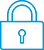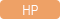cancel
Showing results for
Search instead for
Did you mean:It has been a while since anyone has replied. Simply ask a new question if you would like to start the discussion again.New member
2 1 0 0
Message 1 of 4
610
Flag Post

Solved!

# How do I input the calculation if the compounds per year differ from the payments per year?HP Recommended
HP 10bii +
Microsoft Windows 10 (64-bit)

How would I input this question into my calculator? My class is using a different calculator, please help

How much money should Mark invest today in a fund that earns interest at 4.74% compounded quarterly, if he wants to receive \$4,250 at the end of every 6 months for the next 3 years?

Tags (2)
3 REPLIES 3Level 6
252 251 42 77
Message 2 of 4
Flag PostHP Recommended

Hello,

If I understand your problem correctly, the exercise is:

investment of X

interest = 4.75% every 3 months (I am assuming that this is an effective interest, nor nominal)

payements = 4250 every 6 months

duration = 3 years (6 periods)

enter P/YR=1 (this will simplify the calculations as we will not have to transform the interest from effective to nominal)

enter I%= (1.0475²-1)*100. What we are doing here is calculating the interest for 6 month. assuming that the interest rate is effective, we need to square (compound) the interest rate over a 2 month period to make it work...

enter N=6

make sure the other registers are at 0

type of PV to get the present value = X

Another way to do it, maybe less convoluted would be to use Cash flows to calculate the NPV of an investement with alternatives Ni of 4250 and 0

Cyrille

I am an HP Employee
Tags (2)Level 6
305 297 32 76
Message 3 of 4
Flag PostHP Recommended

I think you will have to read pages 80 and 81 of the manual:

Compounding and Payment Periods Differ.

http://h10032.www1.hp.com/ctg/Manual/c02989763.pdf

Use this to calculate the adjusted interest rate, and next store:

Payment = PMT = 4250, maybe a minus sign.

Number of payments = N = 6

Future value = FV = 0

And solve for present value = PVNew member
2 1 0 0
Message 4 of 4
Flag PostHP Recommended

Thank you for the help.  The calculator that my classmates are using has a c/y function, I guess this one doesn't.

† The opinions expressed above are the personal opinions of the authors, not of HP. By using this site, you accept the Terms of Use and Rules of Participation